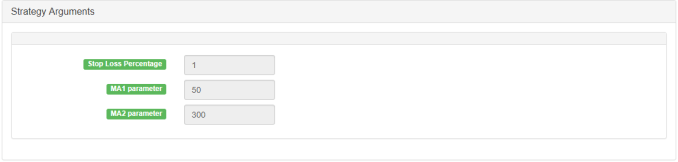# 均线与RSI相对强弱指数组合策略的运用

Author: , Created: 2019-07-31 14:28:28, Updated: 2019-08-01 18:02:44

## RSI指标的原理与运用

RSI是通过比较上涨天数与下降天数的强弱来衡量一个交易标的对自身表现的基本指标。该数字是计算出来的，其范围在0到100之间。高于70的读数被认为是看涨的，而低于30的读数则表示看跌。

## 相对强弱指数公式

RSI由J.Welles Wilder开发，并于1978年6月在他的“技术交易系统新概念”一书中详述。对于所有硬核技术分析人员，下面是相对强度指数公式的例子。

RSI的默认设置为14天，因此您可以根据以下公式计算：

**相对强度 = 1.25（过去13根K线平均涨幅）+ 0.25（当前涨幅）/（0.75（过去13根K线平均跌幅）+ 0（当前跌幅））

RSI = 100 - [100 /（1+2）] = 66.67**

## 在发明者量化平台编写和运用均线加RSI策略

• 策略名称：均线与RSI相对强弱指数组合策略
• 周期：15分钟，30分钟，等等
• 支持：商品期货，数字货币``````MA 1, formula: MA1 ^^ EMA (C, N1);
MA 2, formula: MA2 ^^ EMA (C, N2);
``````

``````RSI, formula:
RSIVALUE:SMA(MAX(CLOSE-REF(CLOSE,1),0),LENGTH,1)/SMA(ABS(CLOSE-REF(CLOSE,1)),LENGTH,1)*100;
````````````MA1^^EMA(C,N1);
MA2^^EMA(C,N2);

LENGTH:=9;
OVERBOUGHT:=70;
OVERSOLD:=100-OVERBOUGHT;
RSIVALUE:SMA(MAX(CLOSE-REF(CLOSE,1),0),LENGTH,1)/SMA(ABS(CLOSE-REF(CLOSE,1)),LENGTH,1)*100;
BUYK:=BKVOL=0 AND BARPOS>N2 AND MA1>MA2 AND C>MAX(MA1,MA2) AND CROSSUP(RSIVALUE,OVERBOUGHT);
SELLK:=SKVOL=0 AND BARPOS>N2 AND MA1<MA2 AND C<MIN(MA1,MA2) AND CROSSDOWN(RSIVALUE,OVERSOLD);
SELLY:=MA1<MA2 AND C>BKPRICE*(1+SLOSS*0.01);
SELLS:=C<BKPRICE*(1-SLOSS*0.01);